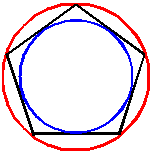Cleave Books
The Regular Polygons Calculator
 Show values to . . . 3 4 5 6 7 8 9 significant figures.
 number of edges(3 to 1000) length of edge unitsarea square units in-radius units circum-radius units
Remember: Appropriate units need to be attached.
Very large and very small numbers appear in e-Format.
Unvalued zeros on all numbers have been suppressed.
A note on Format and Accuracy is available.

A regular polygon is one which has all its edges the same length, and all its vertex angles the same size.
There are two particular circles associated with any regular polygon.
First is the circumcircle which is the circle drawn around the outside of the polygon so as to touch all its vertices (or corners). This circle is usually important when constructing a polygon.
Its radius is given here as the CIRCUM-radius.
Second is the incircle which is the circle drawn around the inside of the polygon so as to touch all its edges. Its size is given here by the IN-radius.
In the diagram, the CIRCUMcircle is RED; the INcircle is BLUE.
If the perimeter is needed, it is
number of edges × length of one edge

Polygons are named by the number of edges (or vertices) they have. Some of the most commonly used names are
 Edges Name 3 triangle 4 quadrilateral 5 pentagon 6 hexagon 7 heptagon 8 octagon 9 nonagon 10 decagon 11 undecagon 12 dodecagon

Go to Cleave Books main Index